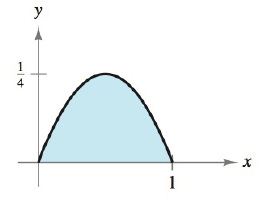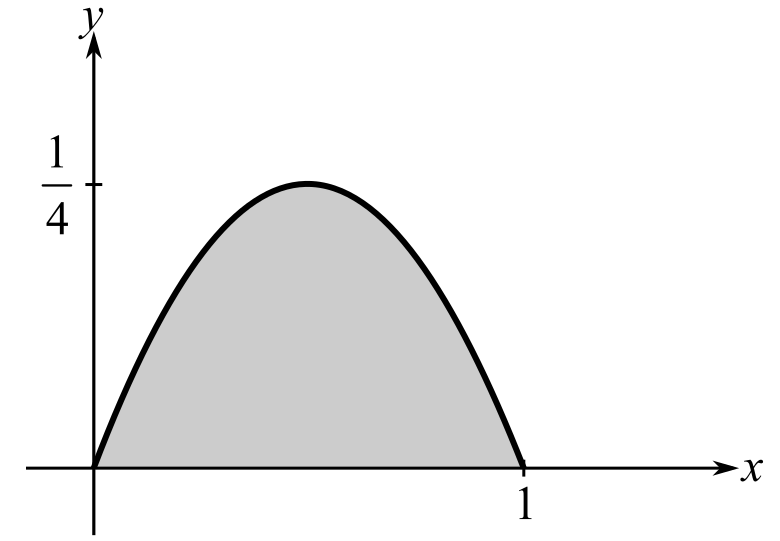Chapter 5.4, Problem 9E### Calculus: An Applied Approach (Min...

10th Edition
Ron Larson
ISBN: 9781305860919

#### Solutions

Chapter
Section### Calculus: An Applied Approach (Min...

10th Edition
Ron Larson
ISBN: 9781305860919
Textbook Problem
1 views

# Finding Area by the Fundamental Theorem In Exercises 9-16, find the area of the region. See Example 2. y = x − x 2To determine

To calculate: The area of the region y=xx2 in the provided figure.Explanation

Given Information:

The function is y=xx2 and the provided figure is:

Formula used:

The fundamental theorem of calculus states that,

If f is integrable on interval [a,b] then abf(x)dx=F(b)F(a).

The integration formula is xndx=xn+1n+1+C, where C is the constant.

Calculation:

Consider the given figure.

To find the area of the region, fundamental theorem of calculus can be used.

Integrate the function from 0 to 1 as shown below,

01(xx2)dx

Now find antiderivative by applying the integration formula xndx=xn+1n+1+C

### Still sussing out bartleby?

Check out a sample textbook solution.

See a sample solution

#### The Solution to Your Study Problems

Bartleby provides explanations to thousands of textbook problems written by our experts, many with advanced degrees!

Get Started

#### Find the general indefinite integral. (1+rr)2dr

Single Variable Calculus: Early Transcendentals, Volume I

#### In Exercises 49-62, find the indicated limit, if it exists. 56. limx2x+2x2

Applied Calculus for the Managerial, Life, and Social Sciences: A Brief Approach

#### Multiply:

Mathematical Applications for the Management, Life, and Social Sciences

#### For

Study Guide for Stewart's Multivariable Calculus, 8th

#### limx37x+65x12 = ______. a) 12 b) 75 c) 9 d) 4

Study Guide for Stewart's Single Variable Calculus: Early Transcendentals, 8th